## Wednesday, January 18, 2017

### Supervised Machine Learning with Python

titanic_notebook_II

## Introduction¶

If you are just getting started with Machine Learning and Data Science finding a solid starting point can often be a challenge. With so many online educational opportunities, entry-level and advanced books and blogs on the subject as well as open source projects supporting numerous aspects of AI and Machine Learning, finding a starting point that provides a good foundation on general concepts as well as providing plenty of practical code, can be a challenge.
One excellent starting point and a solid source of information and collaboration on Machine Learning and Data Science is Kaggle.com. On this site, individuals and teams compete to solve interesting problems using Machine Learning. The winners of these competitions gain not only bragging rights but also the potential to win significant cash prizes, with some prizes in excess of \$250,000 US! In addition to cash prizes some competitions are for job opportunities in companies like Facebook, Allstate, Airbnb. _(Update: Kaggle in January of 2017 released a \$1,000,000 competition to develop lung cancer detection algorithms)_
In addition to the reward competitions Kaggle.com also offers a graceful introduction to Supervised Machine Learning through a getting started competition with a number of excellent tutorials. In this competition, you must create a machine-learning model that will predict who will perish and who will survive the infamous shipwreck of the RMS Titanic.
In this paper, we will explore a practical hands-on approach to creating a machine-learning model using Python that can solve this problem. With an instance of Anaconda or using a Kaggle Kernel you will be able to execute the code provided and produce a model that will be very competitive in the Kaggle competition. (As of this writing 1/13/17 the Machine Learning model generated from the code provided places in the top 11% on the Kaggle public leaderboard.) Once you understand the methodology and code, further optimizations from your inputs could bring the model even higher!

### Process¶

Before jumping in, let us take a high-level overview and provide a systematic method to creating our model. Broadly speaking within machine-learning there are three types of learning. These are Supervised, Unsupervised and Reinforcement learning. We will be focusing on the first Supervised learning.
Supervised learning is an algorithm in which both the inputs and outputs (aka labels) are provided for a subset of all possibilities during training. Based on this training data, the algorithm must generalize such that it is able to correctly respond to new inputs that have not been previously evaluated. That is the algorithm is expected to produce correct output for inputs that weren’t encountered during training.
The below diagram provides a high-level pictorial: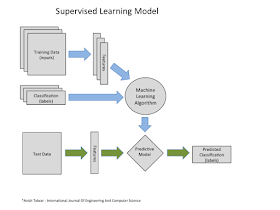In any supervised machine learning process, we follow the methodology below.
1. Load and analyze the data
2. Clean and impute missing data
3. Feature engineering
4. Select Prediction algorithms and parameters
5. Model generation and validation
6. Repeat as needed to optimize model
In creating this document I referenced a number of helpful Kernels and worked through the below-suggested tutorials:

## Load and analyze the data¶

Our first task is to load and analyze the data which can be found here. Our initial analysis should look at the quantity of the data as well as checking the quality and completeness of the data. The initial review should additionally give us an opportunity to make some basic assumptions that could be useful for our learning algorithm.
The below code tells us that we have data for 1,309 passengers and 891 training samples complete with labels (survived or perished). Additionally, we can see we are missing data for some records which we will impute in the next step.
You can find and study some additional background information on our subject here at the encyclopedia titanica We will need to have a solid understanding of our data if we are going to train our model to make accurate predictions.
Data from encyclopedia-titanica.org
1,496 Victims
712 Survivors
--------------
2,208 Total
import pandas as pd
import numpy as np
import re

import matplotlib.pyplot as plt
from numpy.random import rand
from sklearn.ensemble import RandomForestClassifier, ExtraTreesRegressor
from sklearn import tree

# Load the train and test datasets to create two DataFrames
base_url = "./input"
train_url = base_url + "/train.csv"
train_data = pd.read_csv(train_url)

test_url = base_url + "/test.csv"
test_data = pd.read_csv(test_url)

# Combine test and training to facilitate cleanup and pre-processing
full_data = pd.concat([train_data, test_data], axis=0)

print ("Training data (rows, columns) {}\n".format(train_data.shape))
print ("Test     data (rows, columns) {}\n".format(test_data.shape))
print ("Full     data (rows, columns) {}\n".format(full_data.shape))

# Lets see how we are doing with missing values
print("Full data missing values \n{}\n".format(full_data.isnull().sum()))

Training data (rows, columns) (891, 12)

Test     data (rows, columns) (418, 11)

Full     data (rows, columns) (1309, 12)

Full data missing values
Age             263
Cabin          1014
Embarked          2
Fare              1
Name              0
Parch             0
PassengerId       0
Pclass            0
Sex               0
SibSp             0
Survived        418
Ticket            0
dtype: int64



## Clean and impute missing data¶

In this section, we will impute missing data by making some logical assumptions about what these data values mostly are. We will also evaluate a little more closely the data focusing on how it represents the real world. For example, the Cabin field seems to contain the "Deck" of the ship as well as the "Cabin" number. This could be important in calculating survival as someone in a lower deck may have had more difficulty getting to the top of the ship to escape.

### Evaluate Embarked, Cabin, Fare & Ticket¶

This section looks specifically at Embarked, Cabin, Fare and Ticket fields and imputes data where necessary.
Note: Many of the algorithms used later will behave poorly or completely fail if null data is presented. Hence our analysis of the data will not only help provide improved insights it assures the classifier algorithms do not fail on null data.
# Remove Warnings from Pandas
pd.options.mode.chained_assignment = None  # default='warn'

# Lets fill the missing 'Embarked' values with the most occurred value, which is "S".
# 72.5% of people left from Southampton.
print("Embarked")
print(full_data["Embarked"].value_counts(),"\n")

full_data.Embarked.fillna('S', inplace=True)

# There is only one missing 'Fare' and its in the test dataset
# Let's just go ahead and fill the fare value in with the median fare
full_data.Fare.fillna(full_data.Fare.median(), inplace=True)

# Lets take a look at Cabins...
# It looks like 77% and 78% of these fields are empty.
# But just going to map and see what happens
print("Null Cabins in training {:2.2f}%".format(
(1-(train_data["Cabin"].value_counts().sum() / len(train_data["Cabin"])))*100))
print("Null Cabins in test {:.4f}".format(
(1-(test_data["Cabin"].value_counts().sum() / len(test_data["Cabin"])))*100))

# Looking at the data it may be better to pull the Deck from the Cabin
# but just starting here
print()
print(train_data["Cabin"].value_counts().head(10))

# Simple function returns the value of cabin if found or "None" if it is not found
def clean_cabin(x):
try:
return x
except TypeError:
return "None"

# Update Cabin replacing nulls with "None"
full_data.Cabin = full_data.Cabin.apply(clean_cabin)

# Parsing the Ticket values seems a bit confusing
# and I'm not sure what we can gain from this field
print()
print("Ticket")
print(full_data["Ticket"].value_counts().head(),"\n")

Embarked
S    914
C    270
Q    123
Name: Embarked, dtype: int64

Null Cabins in training 77.10%
Null Cabins in test 78.2297

B96 B98            4
G6                 4
C23 C25 C27        4
C22 C26            3
D                  3
F2                 3
F33                3
E101               3
C2                 2
B57 B59 B63 B66    2
Name: Cabin, dtype: int64

Ticket
CA. 2343        11
CA 2144          8
1601             8
3101295          7
S.O.C. 14879     7
Name: Ticket, dtype: int64



### Normalize Data for use with Classification tools (Sex, Embarked, & Cabin)¶

Next, we create some additional dummy variables.
Dummy variables will create new fields in our model and these fields will be represented with values of 0 or 1. They will convert qualitative variables (gender, embarked, cabin, etc.) to a dummy independent variables. When our classifier receives an observation with a value of 0 this will cause that variable to have no role in influencing the dependent variable (survive, perish), while when the dummy takes on a value 1 it acts to have some effect on the dependent varibale.
The following links provide reading with additional details.
# Create categories for Sex, Embarked, and Cabin
full_data = pd.concat([full_data, pd.get_dummies(full_data['Sex'], prefix='Sex')],
axis=1)

full_data = pd.concat([full_data, pd.get_dummies(full_data['Embarked'],
prefix='Embarked')], axis=1)

full_data = pd.concat([full_data, pd.get_dummies(full_data['Cabin'],
prefix='Cabin')], axis=1)


## Feature engineering¶

With our data loaded, analyzed and cleaned our next step which many consider the most important for creating a strong predictive model is feature engineering.
Feature engineering is the process of using domain knowledge of the data to create features that make machine learning algorithms work.

### Derive some new features from the Name field¶

From the name field "Name" we can derive some addition fields that may help in determining survival. Below we will derive "Last Name", "Last Name Count" and "Title".
Our assumption being that if people had the same last name they may be related and if many related people were on the ship they may have tried to find each other before departing. This time spent looking for relatives may have been costly and reduced their likelihood to survive.
Another assumption on title might be those people of privilege or rank, were more likely to survive. "Sir", "Rev", "Countess", etc. We can also use the title as a factor for gender and age. So if the old adage "Women and Children first" is true "Ms" and "Mrs" may be more likely to survive while "Mr" would more likely perish.
# Extract the title from the name
def get_title(name):
index_comma = name.index(',') + 2
title = name[index_comma:]
index_space = title.index('.') + 1
title = title[0:index_space]
return title

# Helper method to show unique_titles
unique_titles = {}
def get_unique_titles(name):
title = get_title(name)
if title in unique_titles:
unique_titles[title] += 1
else:
unique_titles[title] = 1

# Uncomment to show the unique titles in the data set
#full_data["Name"].apply(get_unique_titles)
#print(unique_titles)

# Upon review of the unique titles we consolidate on the below mappings as optimal
def map_title(name):
title = get_title(name)
#should add no key found exception
title_mapping = {"Mr.": 1, "Miss.": 2, "Ms.": 10, "Mrs.": 3, "Master.": 4, "Dr.": 5,
"Rev.": 6, "Major.": 7, "Col.": 7, "Don.": 7, "Sir.": 7, "Capt.": 7,
"Mlle.": 8, "Mme.": 8, "Dona.": 9, "Lady.": 9, "the Countess.": 9,
"Jonkheer.": 9}
return title_mapping[title]

# Create a new field with a Title
full_data["Title"] = full_data["Name"].apply(map_title)

# Extract the last name from the title
def get_last_name(name):
index_comma = name.index(',')
last_name = name[0:index_comma:]
#print(last_name)
return last_name

# Helper method to show unique_last_names
unique_last_names = {}
def get_unique_last_names(name):
last_name = get_last_name(name)
if last_name in unique_last_names:
unique_last_names[last_name] += 1
else:
unique_last_names[last_name] = 1

# Create a new field with last names
full_data["LastName"] = full_data["Name"].apply(get_last_name)

# Create a category by grouping like last names
full_data["Name"].apply(get_unique_last_names)
full_data["LastNameCount"] = full_data["Name"].apply(
lambda x: unique_last_names[get_last_name(x)])


### Impute missing Ages¶

You will recall that we have 263 missing ages. And since it is likely that age plays a strong role in the likelihood of survival we impute the missing ages with a couple of different techniques and then settle on the one that provides the best results.
The first method finds the median age for a person with a given title "Mr", "Ms", "Miss", "Master" (Master was a title given to young boys) and substitutes null values with this median age.
The second method which is a bit more advanced does a regression analysis on age given a number of distinct features and provides and average age based on the occurrence of these features. For example, we may assume that given the "Fare" as a feature that a child's tickets would have been less expensive than and adults tickets. Or that it is more likely that there were more older (and richer) people in first class that second class.
For us to know the exact details of these feature nuances is not necessary as the Regressor will tease out the statistics without additional input on our part.
# To set the missing ages we will find the median age for the person's title and use that
# as the age of the person
def map_missing_ages1(df):
avg_title_age = {}
# Find median age for all non null passengers
avg_age_all= df['Age'].dropna().median()
# Iterate all the titles and set a median age for each title
for title in range(1,11):
avg_age = df['Age'][(df["Title"] == title)].dropna().median()
#If the average age is null for a title default back to average for all passengers
if pd.isnull(avg_age):
avg_age = avg_age_all
avg_title_age[title] = avg_age

# Now that we have a list with average age by title
# we apply it to all our null passengers
for title in range(1,11):
# print("title code:",title," avg age:",avg_title_age[title])
df["Age"][(df["Title"] == title) & df["Age"].isnull()] = avg_title_age[title]

# Set the  missing ages by creating a classifier based on the below criteria
def map_missing_ages2(df):
feature_list = [
"Fare",
"Pclass",
"Parch",
"SibSp",
"Title",
"Sex_female",
"Sex_male",
"Embarked_C",
"Embarked_Q",
"Embarked_S"
]

etr = ExtraTreesRegressor(n_estimators=200,random_state = 42)

train = df.loc[df.Age.notnull(),feature_list]
target = df.loc[df.Age.notnull(),['Age']]

test = df.loc[df.Age.isnull(),feature_list]
etr.fit(train,np.ravel(target))

age_preds = etr.predict(test)
df.loc[df.Age.isnull(),['Age']] = age_preds

# After testing both techniques we are getting better results with the second method
# map_missing_ages1(full_data)
map_missing_ages2(full_data)


### Creating a Fare Category¶

Creating a category for fare is often a better predictor than using the Fare Values alone. That is if we group fares into groups i.e free, cheap, medium price, expensive, etc. This may "generalize better" than the specific individual fare prices, improving the overall quality of predictions.
Using to many feature with fine-grained data can lead to "overfitting" the data to the training set. When this happens the model will do very well on predictions with the training data but will not generalize as well to data that it has not seen before.
# Exploring some of the fare data looking for patterns
# create a Fare Category grouping fare prices

full_data["FareCat"]=0
r=0
for f in range(20,220,20):
full_data["FareCat"][(full_data["Fare"] >= r) & (full_data["Fare"] < f)] = f
print("f >= {} & f < {}".format(r,f))
r=f

full_data["FareCat"][(full_data["Fare"] >= 200)] = 200

#################################################
# Plot the Fare Category
fig, (axis1) = plt.subplots(nrows=1, ncols=1, figsize=(5, 3))

survived = full_data['FareCat'][train_data['Survived']==1].value_counts().sort_index()
died =     full_data['FareCat'][train_data['Survived']==0].value_counts().sort_index()

width = 0.30
x_pos = np.arange(len(survived))

axis1.bar(x_pos, survived, width, color='b', label='Survived')
axis1.bar(x_pos + width, died, width, color='r', label='Died')
axis1.set_xlabel('Fare', fontsize=12)
axis1.set_ylabel('Number of people', fontsize=10)
axis1.legend(loc="upper right", fontsize="xx-small",
ncol=2, shadow=True, title="Legend")
axis1.yaxis.grid(True)

plt.show()

##########################################################
fare_w_0_cost = full_data[(full_data["Fare"]==0.0)]
print(fare_w_0_cost.loc[:,['Survived','Name','Sex','Age','Fare']])

f >= 0 & f < 20
f >= 20 & f < 40
f >= 40 & f < 60
f >= 60 & f < 80
f >= 80 & f < 100
f >= 100 & f < 120
f >= 120 & f < 140
f >= 140 & f < 160
f >= 160 & f < 180
f >= 180 & f < 200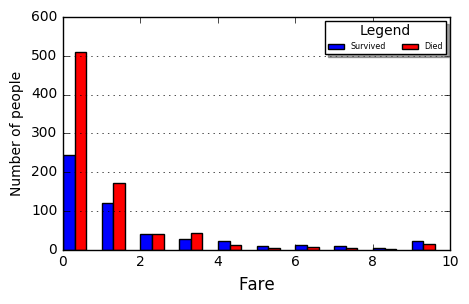Survived                                   Name   Sex        Age  Fare
179       0.0                    Leonard, Mr. Lionel  male  36.000000   0.0
263       0.0                  Harrison, Mr. William  male  40.000000   0.0
271       1.0           Tornquist, Mr. William Henry  male  25.000000   0.0
277       0.0            Parkes, Mr. Francis "Frank"  male  31.416667   0.0
302       0.0        Johnson, Mr. William Cahoone Jr  male  19.000000   0.0
413       0.0         Cunningham, Mr. Alfred Fleming  male  31.416667   0.0
466       0.0                  Campbell, Mr. William  male  31.416667   0.0
481       0.0       Frost, Mr. Anthony Wood "Archie"  male  31.416667   0.0
597       0.0                    Johnson, Mr. Alfred  male  49.000000   0.0
633       0.0          Parr, Mr. William Henry Marsh  male  42.666667   0.0
674       0.0             Watson, Mr. Ennis Hastings  male  31.416667   0.0
732       0.0                   Knight, Mr. Robert J  male  31.416667   0.0
806       0.0                 Andrews, Mr. Thomas Jr  male  39.000000   0.0
815       0.0                       Fry, Mr. Richard  male  42.666667   0.0
822       0.0        Reuchlin, Jonkheer. John George  male  38.000000   0.0
266       NaN  Chisholm, Mr. Roderick Robert Crispin  male  42.666667   0.0
372       NaN                Ismay, Mr. Joseph Bruce  male  49.000000   0.0


### Visualization of key features¶

Providing Visualizations of our features helps us to determine what features are more or less likely to be influential factors on survival or death. The more we know about our data and how it influences the results the better out predictive model will be.
In this section, we look at Gender, Passenger Class, Embarked, Age, and Fare.
From our visualizations we can derive the following:
1. Looking at gender it is clear that far more women survive than men making the adage "women and children first" likely true for the passengers of the Titanic.
2. Older people (over 65) are more likely to die than younger people (younger than 10)
3. People in Thrid Class are more likely to die than those in First Class. This may be because Thrid Class cabins were deep down in the lower levels of the ship whereas First and Second Class were closer to the top and therefore closer to the lifeboats and safety.
4. It's interesting that more people from Southampton died than other departing ports but this could be because the ship's crew boarded in Southampton and may have been more likely to have stayed on board to help passengers.
fig, ((axis1, axis2),(axis3, axis4)) = plt.subplots(nrows=2, ncols=2, figsize=(9, 7))

###########################################################
pclass_survived = train_data['Pclass'][train_data['Survived']==1].value_counts().sort_index()
pclass_died =     train_data['Pclass'][train_data['Survived']==0].value_counts().sort_index()

width = 0.30
x_pos = np.arange(len(pclass_survived))

axis1.bar(x_pos,pclass_survived, width, color='blue', label='Survived')
axis1.bar(x_pos + width, pclass_died, width, color='red', label='Died')
axis1.set_xlabel('Passenger Classes', fontsize=12)
axis1.set_ylabel('Number of people', fontsize=10)
axis1.legend(loc='upper center')
axis1.set_xticklabels(('','First Class','','Second Class','','Third Class'))
axis1.yaxis.grid(True)

###########################################################
embrk_survived = train_data['Embarked'][train_data['Survived']==1].value_counts().sort_index()
embrk_died     = train_data['Embarked'][train_data['Survived']==0].value_counts().sort_index()

#print(embarked_died)
#print(embarked_survived)
x_pos = np.arange(len(embrk_survived))
axis2.bar(x_pos,embrk_survived, width, color='blue', label='Survived')
axis2.bar(x_pos + width, embrk_died, width, color='red', label='Died')
axis2.set_xlabel('Embarked From', fontsize=12)
axis2.set_ylabel('Number of people', fontsize=10)
axis2.legend(loc='upper center')
axis2.set_xticklabels(('','Cherbourg','','Queenstown','','Southamton'))
axis2.yaxis.grid(True)

###########################################################
# Age fill has an interesting spike based on the above fill of empty ages
age_survived = train_data['Age'][train_data['Survived']==1].value_counts().sort_index()
age_died     = train_data['Age'][train_data['Survived']==0].value_counts().sort_index()

minAge, maxAge = min(train_data.Age), max(train_data.Age)
bins = np.linspace(minAge, maxAge, 100)

# You can squash the distribution with a log function but I prefer to see the outliers
#axis3.bar(np.arange(len(age_survived)),np.log10(age_survived),
#                                          color='blue', label='Survived')
#axis3.bar(np.arange(len(age_died)), -np.log10(age_died),
#                                          color='red', label='Died')

axis3.bar(np.arange(len(age_survived)), age_survived, color='blue', label='Survived')
axis3.bar(np.arange(len(age_died)), -(age_died), color='red', label='Died')
#axis3.set_yticks(range(-3,4), (10**abs(k) for k in range(-3,4)))
axis3.legend(loc='upper right',fontsize="x-small")
axis3.set_xlabel('Age', fontsize=12)
axis3.set_ylabel('Number of people', fontsize=10)

###########################################################
# Chart Fare by Survived and Perished
fair_survived = train_data['Fare'][train_data['Survived']==1].value_counts().sort_index()
fair_died     = train_data['Fare'][train_data['Survived']==0].value_counts().sort_index()

minAge, maxAge = min(train_data.Age), max(train_data.Age)
bins = np.linspace(minAge, maxAge, 100)

axis4.bar(np.arange(len(fair_survived)), fair_survived, color='blue', label='Survived')
axis4.bar(np.arange(len(fair_died)), -(fair_died), color='red', label='Died')
#axis4.set_yticks(range(-3,4), (10**abs(k) for k in range(-3,4)))
axis4.legend(loc='upper right',fontsize="x-small")
axis4.set_xlabel('Fare', fontsize=12)
axis4.set_ylabel('Number of people', fontsize=10)

plt.show()

###########################################################
fig, (axis1) = plt.subplots(nrows=1, ncols=1, figsize=(5, 3))

survived = train_data['Sex'][train_data['Survived']==1].value_counts().sort_index()
died =     train_data['Sex'][train_data['Survived']==0].value_counts().sort_index()

width = 0.30
x_pos = np.arange(len(survived))

axis1.bar(x_pos, survived, width, color='b', label='Survived')
axis1.bar(x_pos + width, died, width, color='r', label='Died')
axis1.set_xlabel('Gender', fontsize=12)
axis1.set_ylabel('Number of people', fontsize=10)
axis1.set_xticklabels(('','Females','','','','','Males'))
axis1.legend(loc="upper left", fontsize="xx-small",
ncol=2, shadow=True, title="Legend")
axis1.yaxis.grid(True)

plt.show()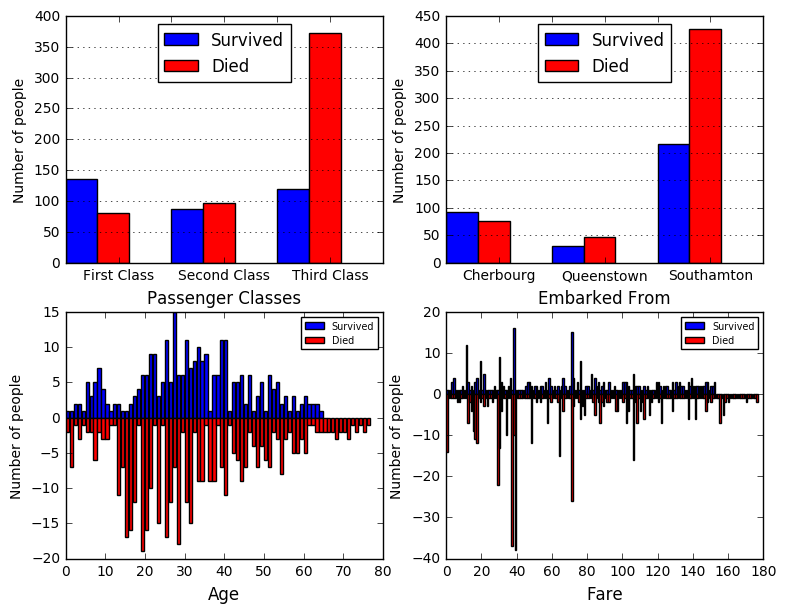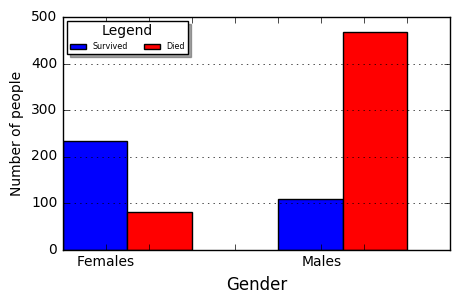### Creating additional features¶

In this section, we will dig a bit deeper and use our domain knowledge to further refine and combine the given data into features with higher predictive capabilities.
From our analysis Children seem to have had a higher survival rate so we create a specific feature to represent this.
If "Women and Children first" is a good predictor alone then being a mother may be an even better predictor so we create a feature for this.
Creating a category feature for family size may provide some good additional predictive insights.
Next, we take the log2 of Age and Fare to "normalize" the data distribution. You will recall from the visualizations provided above that the age and fare data provided, loosely follow a "normal distribution". Statistically, we would expect a closer representation to "normal" if we had more data. Taking the log creates a distribution closer to "normal" and therefore may have better predictive characteristics on none trained data inputs.
Next, we create some features that combine data and tally survival percentages based on these combinations. For Example, the feature Class*MWC looks at Males, Females and Children and tallies their likelihood to survive based which class (1st, 2nd, or 3rd) they were on. Combining features in this manner can reduce noise in the model and improve the predictive capabilities.
# Lets do some feature engineering

# Assign 1 to passengers under 14, 0 to those 14 or older.
full_data["Child"] = 0
full_data["Child"][full_data["Age"] < 14] = 1

# Create a Mother field (It seems Mothers had a pretty high survival rate)
# Note that Title "Miss." = 2 in our mappings
full_data["Mother"] = 0
full_data["Mother"][(full_data["Parch"] > 0) & (full_data["Age"] > 18) &
(full_data["Sex"] == 'female') & (full_data["Title"] != 2)] = 1

full_data["FamilySize"] = full_data["SibSp"] + full_data["Parch"]

# Create a Family category none, small, large
full_data["FamilyCat"] = 0
full_data["FamilyCat"][ (full_data["Parch"] + full_data["SibSp"]) == 0] = 0
full_data["FamilyCat"][((full_data["Parch"] + full_data["SibSp"]) > 0) &
((full_data["Parch"] + full_data["SibSp"]) <= 3)] = 1
full_data["FamilyCat"][ (full_data["Parch"] + full_data["SibSp"]) > 3 ] = 2

full_data["SingleMale"] = 0 #0 -- Other ends up being females
full_data["SingleMale"][((full_data["Parch"] + full_data["SibSp"]) == 0) &
(full_data["Sex"] == 'male')] = 2
full_data["SingleMale"][((full_data["Parch"] + full_data["SibSp"]) >  0) &
(full_data["Sex"] == 'male')] = 1

full_data["AdultFemale"] = 0
full_data["AdultFemale"][(full_data["Age"] > 18) & (full_data["Sex"] == 'female')] = 1

full_data["AdultMale"] = 0
full_data["AdultMale"][(full_data["Age"] > 18) & (full_data["Sex"] == 'male')] = 1

full_data["PclassXAge"]= full_data["Pclass"] * full_data["Age"]
full_data["FareDivPclass"]= full_data["Fare"] / full_data["Pclass"]

import math

full_data["FareLog"] = full_data["Fare"].apply(lambda x: 0 if x == 0 else math.log2(x))
full_data["AgeLog"] = full_data["Age"].apply(lambda x: 0 if x == 0 else math.log2(x))

train_data = full_data.iloc[:891,:]
##########################################################
full_data["Class*MWC"]=0
# Return the likelihood to survive based on class and gender
def survive_percentage_class(pclass,gender):
if gender == 'child':
x = train_data["Survived"][(train_data["Pclass"] == pclass) &
(train_data["Child"] == 1 )]
else:
x = train_data["Survived"][(train_data["Pclass"] == pclass) &
(train_data["Sex"] == gender ) &
(train_data["Child"] != 1 )]

y = x.value_counts(normalize=True).sort_index()
if len(y) >1:
y = y
else:
y = 1.0
#print(int(round(y*100)) )
return int(round(y*100))

# Iterate pclasses and gender and create new feature based on likelihood to survive
for gender in ['female','male','child']:
#print("")
for pclass in [1,2,3]:
if gender == 'child':
full_data["Class*MWC"][(full_data["Pclass"] == pclass) &
(full_data["Child"] == 1 )] = survive_percentage_class(pclass,gender)
else:
full_data["Class*MWC"][(full_data["Pclass"] == pclass) &
(full_data["Sex"] == gender )] = survive_percentage_class(pclass,gender)

##########################################################
full_data["Fare*MWC"]=0
# Return the likelihood to survive based on Fare and gender
def survive_percentage_fare(fare_cat,gender):
if gender == 'child':
x = train_data["Survived"][(train_data["FareCat"] == fare_cat) &
(train_data["Child"] == 1 )]
else:
x = train_data["Survived"][(train_data["FareCat"] == fare_cat) &
(train_data["Sex"] == gender ) &
(train_data["Child"] != 1 )]

y = x.value_counts(normalize=True).sort_index()
if len(y) >1:
y = y
else:
y = 1.0
#print(int(round(y*100)) )
return int(round(y*100))

# Iterate Fare category and gender and create new feature based on likelihood to survive
for gender in ['female','male','child']:
#print("")
for fare_cat in range(20,220,20):
if gender == 'child':
full_data["Fare*MWC"][(full_data["FareCat"] == fare_cat) &
(full_data["Child"] == 1 )] = survive_percentage_fare(fare_cat,gender)
else:
full_data["Fare*MWC"][(full_data["FareCat"] == fare_cat) &
(full_data["Sex"] == gender )] = survive_percentage_fare(fare_cat,gender)

##########################################################

full_data["Compartment"]=0
# Return the likelihood to survive based compartment
def survive_percentage_compartment(cat):
x = train_data["Survived"][(train_data["Cabin"] == cat)]
y = x.value_counts(normalize=True).sort_index()
if len(y) >1:
y = y
else:
y = 1.0
#print("cat:",cat,"y:\n",x.value_counts(normalize=True).sort_index())
#print("y:",int(round(y*100)) )
return int(round(y*100))

# Iterate Fare category and gender and create new feature based on likelihood to survive
for compartment in ['A','B','C','D','E','F','G','T','N']:
full_data["Compartment"][(full_data["Cabin"] == compartment) ] = \
survive_percentage_compartment(compartment)


### Visualize our new features¶

Let's now take a look at our newly created features and see visually if they are likely to improve our model.
It seems a couple indicators such as not having children increases the likelihood that you will NOT survive! So this one looks helpful.
Title may not be giving us much more information than we can already derive from Gender and Age.
Mother may also not be providing much more insight than Parent which we already have.
# Create some plots of our New Features
fig, ((axis1, axis2),(axis3, axis4)) = plt.subplots(nrows=2, ncols=2, figsize=(9, 7))
train = full_data.iloc[:891,:]

width = 0.30

###########################################################
single_male_survived=train['SingleMale'][train['Survived']==1].value_counts().sort_index()
single_male_died = train['SingleMale'][train['Survived']==0].value_counts().sort_index()

x_pos = np.arange(len(single_male_survived))

axis1.bar(x_pos, single_male_survived, width, color='b', label='Survived')
axis1.bar(x_pos + width, single_male_died, width, color='r', label='Died')
axis1.set_xlabel('Male Marital Status', fontsize=12)
axis1.set_ylabel('Number of people', fontsize=10)
axis1.set_xticklabels(('','Females','','Single Male','','Married Male'))
axis1.legend(loc="upper left", fontsize="xx-small",
ncol=2, shadow=True, title="Legend")

axis1.annotate('Single Males survive \nbetter than Married', xy=(1.2, 100),
xytext=(1, 150), arrowprops=dict(facecolor='black', shrink=0.05),)

###########################################################
mother_survived = train['Mother'][train['Survived']==1].value_counts().sort_index()
mother_died =     train['Mother'][train['Survived']==0].value_counts().sort_index()

x_pos = np.arange(len(mother_survived))

axis2.bar(x_pos, mother_survived, width, color='b', label='Survived')
axis2.bar(x_pos + width, mother_died, width, color='r', label='Died')
axis2.set_xlabel('Mother Status', fontsize=12)
axis2.set_ylabel('Number of people', fontsize=10)
axis2.set_xticklabels(('','All others','','','','','Mothers'))
axis2.legend(loc="upper right", fontsize="xx-small",
ncol=2, shadow=True, title="Legend")

###########################################################
family_survived = train['FamilyCat'][train['Survived']==1].value_counts().sort_index()
family_died =     train['FamilyCat'][train['Survived']==0].value_counts().sort_index()

x_pos = np.arange(len(family_survived))

axis3.bar(x_pos, family_survived, width, color='b', label='Survived')
axis3.bar(x_pos + width, family_died, width, color='r', label='Died')
axis3.set_xlabel('Family Status', fontsize=12)
axis3.set_ylabel('Number of people', fontsize=10)
axis3.set_xticklabels(('','No Kids','','1 to 3 kids','','> 3 Kids',''))
axis3.legend(loc="upper right", fontsize="xx-small",
ncol=2, shadow=True, title="Legend")

###########################################################
title_survived = train['Title'][train['Survived']==1].value_counts().sort_index()
title_died =     train['Title'][train['Survived']==0].value_counts().sort_index()

width = 0.40
x_pos_s = np.arange(len(title_survived))
x_pos_d = np.arange(len(title_died))

axis4.bar(x_pos_s, title_survived, width, color='b', label='Survived')
axis4.bar(x_pos_d + width, title_died, width, color='r', label='Died')
axis4.set_xlabel('Title Status', fontsize=12)
axis4.set_ylabel('Number of people', fontsize=10)
axis4.set_xticklabels(('Mr','Miss','Mrs','Mst','Dr','Rev','Sir','Ml','Lady','Ms'))
axis4.legend(loc="upper right", fontsize="xx-small",
ncol=2, shadow=True, title="Legend")

plt.show()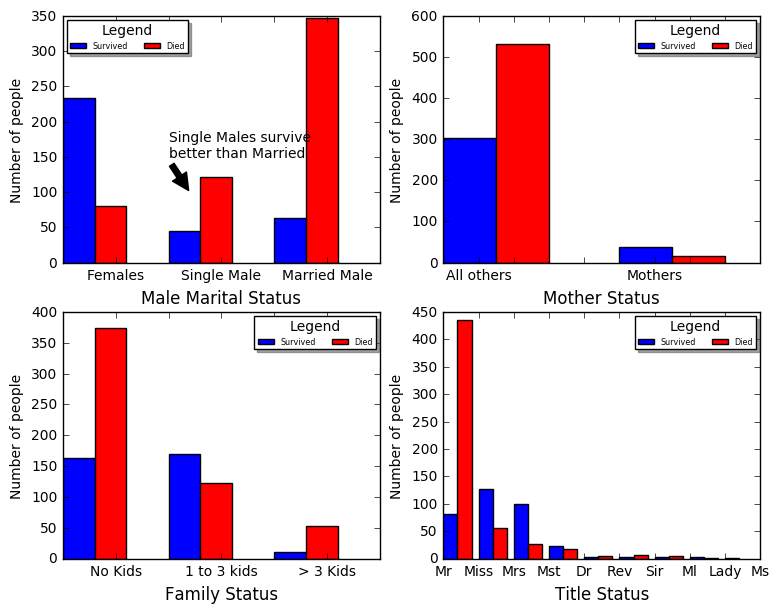### Use Random Forest & Predict¶

After trying a number of other Classifiers we land on Random Forset as it seems to provide the best results. The next section will provide a number of additional classifiers so the reader may try others to explore if they may get better results using different classifiers.
Once we settle on a classifier we can further optimize our model by setting the classifiers parameters. Although there are numerous parameters the ones I have found most helpful are:
n_estimators: The number of trees in the forest.
max_features: Consider max_features features at each split.
max_depth: The maximum depth of the tree. If None, then nodes are expanded until all leaves are pure or until all leaves contain less than min_samples_split samples.
Tuning the Classifier is somewhat of an art as the classifier will generally improve on the training data but at some point will start to "overfit" to the training data and become less capable to predict correctly on new unobserved data. Finding this balance is what produces the best model. In a later section, we will describe how we can further automate the tuning process.
This function also produces the output csv file that is used for submission to Kaggle. Kaggle tests your results against the actual results (Survival or Perish) of the given passengerIds and provides you with your position on the leaderboard. That is how well your model compares to other submitted models. (Using this model you will outperform nearly 90% of other competitors.)
# Setup calassifier and predict

def titanic_predict(feature_list, n_estimators, max_features, max_depth):

train_all      = full_data.iloc[:891,:]
train_features = train_all.loc[:,feature_list]
train_target   = train_all.loc[:,['Survived']]
test_data      = full_data.iloc[891:,:]
test_features  = test_data.loc[:,feature_list]

PassengerId =np.array(test_data["PassengerId"]).astype(int)

#=============================
# Building and fitting the Random forest
forest = RandomForestClassifier(n_estimators=n_estimators,
max_features=max_features,
max_depth=max_depth,
#criterion='entropy',
random_state=42)
forest.fit(train_features, np.ravel(train_target))
forest_pred = forest.predict(test_features)
print("RandomForestClassifier score:",forest.score(train_features, train_target))
#print(my_forest.feature_importances_)
forest_solution = pd.DataFrame(forest_pred, PassengerId,
columns =["Survived"]).astype(int)
forest_solution.to_csv("predict_random_forest.csv", index_label = ["PassengerId"])

# Check that the data frame has 418 entries
print(forest_solution.shape)
return forest_solution, forest

feature_list = [
"Age",
"Fare",
"Pclass",
"Parch",
"SibSp",
"Sex_female",
"Sex_male",
"Embarked_C",
"Embarked_Q",
"Embarked_S",
"Cabin_A",
"Cabin_B",
"Cabin_C",
"PclassXAge",
"FareDivPclass",
"Cabin_D",
"Cabin_E",
"Cabin_F",
"Cabin_G",
"Cabin_None",
"Cabin_T",
"Class*MWC",
"Compartment",
"FareLog",
"FareCat",
"Fare*MWC",
"AgeLog",
"Title",
"Mother",
"FamilySize",
"FamilyCat",
"SingleMale",
"AdultFemale",
"AdultMale",
"LastNameCount",
"Child"
]

my_solution, my_forest = titanic_predict(feature_list,500,None,5)

RandomForestClassifier score: 0.875420875421
(418, 1)


### Other potential classifiers¶

The scikit-learn package provides a number of additional classifiers that are also candidates to provide predictive models for our Titanic problem. Below we configure and test a number of these. From the "score" results you can see that K Nearest Neighbors and Support Vector Machines provide results close to or better than our Random forest Classifier on the training data, but what we have observed is that these models do not generalize as well on the test data and therefore do not perform as well overall.
Additional Classifiers provided
1. Logistic Regression
2. Gaussian Naive Bayes
3. K Nearest Neighbors
4. Support Vector Machines
# Try other classifiers
# None of the below-produced results for me better than the Random Forest
# But I did not try to do much tuning
def other_classifiers(feature_list):
train_all      = full_data.iloc[:891,:]
train_features = train_all.loc[:,feature_list]
train_target   = train_all.loc[:,['Survived']]
test_data      = full_data.iloc[891:,:]
test_features  = test_data.loc[:,feature_list]

PassengerId =np.array(test_data["PassengerId"]).astype(int)

#=============================
# Logistic Regression
from sklearn.linear_model import LogisticRegression
logreg = LogisticRegression()
logreg.fit(train_features, np.ravel(train_target))
logreg_pred = logreg.predict(test_features)
print("LogisticRegression score:",logreg.score(train_features, train_target))
logreg_solution = pd.DataFrame(logreg_pred, PassengerId,
columns =["Survived"]).astype(int)
logreg_solution.to_csv("predict_logistic_regression.csv",
index_label = ["PassengerId"])
#===============================

#===============================
# Gaussian Naive Bayes
from sklearn.naive_bayes import GaussianNB
gaussian = GaussianNB()
gaussian.fit(train_features, np.ravel(train_target))
gaussian_pred = gaussian.predict(test_features)
print("GaussianNB score:",gaussian.score(train_features, train_target))
gaussian_solution=pd.DataFrame(gaussian_pred, PassengerId,
columns= ["Survived"]).astype(int)
gaussian_solution.to_csv("predict_gaussian_nb.csv",
index_label = ["PassengerId"])
#===============================

#===============================
# K Neighbors
from sklearn.neighbors import KNeighborsClassifier
knn = KNeighborsClassifier(n_neighbors = 3)
knn.fit(train_features, np.ravel(train_target))
knn_pred = knn.predict(test_features)
print("KNeighborsClassifier score:",knn.score(train_features, train_target))
knn_solution = pd.DataFrame(knn_pred, PassengerId,
columns = ["Survived"]).astype(int)
knn_solution.to_csv("predict_k_neighbors.csv",
index_label = ["PassengerId"])
#===============================

#===============================
# Support Vector Machines
from sklearn.svm import SVC
svc = SVC()
best_params = {'gamma': 0.015625, 'C': 8192.0, 'kernel': 'rbf'}
#print(best_params)
svc.set_params(**best_params)
svc.verbose=True
svc.fit(train_features, np.ravel(train_target))
svc_pred = svc.predict(test_features)
print("SupportVectorMachine score:",svc.score(train_features, train_target))
svc_solution = pd.DataFrame(svc_pred, PassengerId,
columns = ["Survived"]).astype(int)
svc_solution.to_csv("predict_support_vector_machine.csv",
index_label = ["PassengerId"])
#===============================

#import xgboost as xgb
# Requires additional installation
# not jus pip install

#Uncomment to try other classifiers
other_classifiers(feature_list)

LogisticRegression score: 0.838383838384
GaussianNB score: 0.796857463524
KNeighborsClassifier score: 0.874298540965
[LibSVM]SupportVectorMachine score: 0.978675645342


### Visualize feature importance¶

To help us refine and explore our feature engineering we produce the below function that graphs the relative "importance" of the provided feature. The function additionally aggregates the results of our "dummy fields" into a single value to better represent the dummy field data.
#Graph the importance of the features that have been created.
#Note: Use the categorical_variables variable to aggregate split categories

def graph_feature_importance(model, feature_names, autoscale=True, headroom=0.05,
width=10, summarized_columns=None):

if autoscale:
x_scale = model.feature_importances_.max() + headroom
else:
x_scale = 1

feature_dict = dict(zip(feature_names, model.feature_importances_))

if summarized_columns:
for col_name in summarized_columns:
sum_value=0.0
for i, x in feature_dict.items():
if col_name in i:
sum_value += x
keys_to_remove = [i for i in feature_dict.keys() if col_name in i]
for i in keys_to_remove:
feature_dict.pop(i)

feature_dict[col_name] = sum_value

import numpy as np

#This line below was difficult to figure out!!
fme = np.array(list(feature_dict.values())).flatten()
results = pd.Series(fme, index=feature_dict.keys())
results.sort()
results.plot(kind='barh', figsize=(width,len(results)/4), xlim=(0,x_scale))
plt.show()

categorical_variables = ["Sex", "Cabin","Embarked"]
graph_feature_importance(my_forest,feature_list,summarized_columns=categorical_variables)

/Users/danhiggins/.pyenv/versions/3.5.1/envs/kaggle/lib/python3.5/site-packages/ipykernel/__main__.py:31: FutureWarning: sort is deprecated, use sort_values(inplace=True) for INPLACE sorting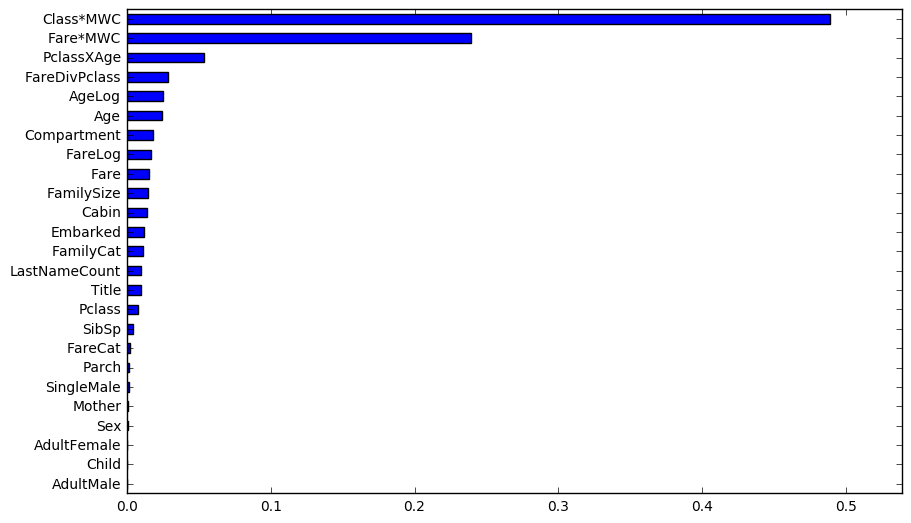### Optimize Classifier Parameters¶

To optimize the model we score our model with the training data and validate against a test data set. The test data score is essentially the score you will receive from Kaggel. (Of the 418 PassengerId's is the model correct in predicting if the particular passenger died or survived.)
Another method of optimizing is cross-validation in this method you would hold out a small subset of the training data and not training our model with this data but instead use it for testing.
# Loop through different options of the Classifier
# Select the best also a good place to put cross-validation test

# score provides a cross-validation
# This code is NOT provided (Left to the read to implement.)
import src.score_titanic_run as score

#n_estimator_options = [30,50,100,200,500,1000,2000]
n_estimator_options = [400,500,1000]
max_features_options = ["auto", None, "sqrt", "log2", 0.9, 0.2, 0.1]
max_features_options = [None]
#max_depth_options = [2,3,4,5,6,7,8,9,10]
max_depth_options = [5,6]
#max_depth_options = [None]
for n_estimators in n_estimator_options:
for max_features in max_features_options:
for max_depth in max_depth_options:
titanic_predict(feature_list, n_estimators, max_features, max_depth)
print("n_estimators=",n_estimators,"max_features=",max_features,
"max_depth=",max_depth)
# Comment out score.run1() if not provided
score.run1()

RandomForestClassifier score: 0.876543209877
(418, 1)
n_estimators= 400 max_features= None max_depth= 5
Data!   Correct  Wrong
Results  327  91

RandomForestClassifier score: 0.897867564534
(418, 1)
n_estimators= 400 max_features= None max_depth= 6
Data!   Correct  Wrong
Results  332  86

RandomForestClassifier score: 0.875420875421
(418, 1)
n_estimators= 500 max_features= None max_depth= 5
Data!   Correct  Wrong
Results  328  90

RandomForestClassifier score: 0.897867564534
(418, 1)
n_estimators= 500 max_features= None max_depth= 6
Data!   Correct  Wrong
Results  333  85

RandomForestClassifier score: 0.87317620651
(418, 1)
n_estimators= 1000 max_features= None max_depth= 5
Data!   Correct  Wrong
Results  331  87

RandomForestClassifier score: 0.901234567901
(418, 1)
n_estimators= 1000 max_features= None max_depth= 6
Data!   Correct  Wrong
Results  333  85



### Another optimization method¶

The scikit-learn package provides a method similar to the above optimization technique. The method is called GridSearchCV and we demonstrate its usage below. However, after evaluating this method with the above we recognize that it does a good job of optimizing the score on the training data but it tends to "overfit".
# The below function helps to optimize your classifier
# allowing you to run a series of tests and see what parameters fit best.
# Although I was excited when I found this the results I found where
# not as good as the Manual process that I am applying above
#
def grid_search():
train_all      = full_data.iloc[:891,:]
train_features = train_all.loc[:,feature_list]
train_target   = train_all.loc[:,['Survived']]
test_data      = full_data.iloc[891:,:]
test_features  = test_data.loc[:,feature_list]

PassengerId =np.array(test_data["PassengerId"]).astype(int)

from sklearn.model_selection import GridSearchCV, StratifiedKFold
n_folds = 10
cv = StratifiedKFold(n_folds)
N_es = [50, 100, 200, 400, 500]
criteria = ['gini', 'entropy']
#
random_forest = RandomForestClassifier()
gscv = GridSearchCV(estimator=random_forest,
param_grid=dict(n_estimators=N_es, criterion=criteria),
n_jobs=1,
cv=list(cv.split(train_features, np.ravel(train_target))),
verbose=2)
gscv.fit(train_features, np.ravel(train_target))
gscv_pred = gscv.predict(test_features)
print("GridSearchCV score:",gscv.score(train_features, train_target))
forest_solution = pd.DataFrame(gscv_pred, PassengerId,
columns = ["Survived"]).astype(int)
forest_solution.to_csv("predict_grid_search.csv",
index_label = ["PassengerId"])

#grid_search()


## Conclusion¶

I hope you have enjoyed working through this Titanic problem. Throughout this paper we developed code that will be useful in solving Supervised Machine Learning Problems. You have learned techniques for imputing missing data, the importance of domain knowledge in feature engineering, techniques for visualization and automating optimization of your model.
You should now be ready to not only tackle and improve upon the Titanic problem with your own submission but you should also be ready to apply your new knowledge to other Supervised Machine Learning Problems.